# GCT解读

## 简介

• 论文标题

Gaussian Context Transformer

• 论文地址

• 论文源码

暂未开源

## 介绍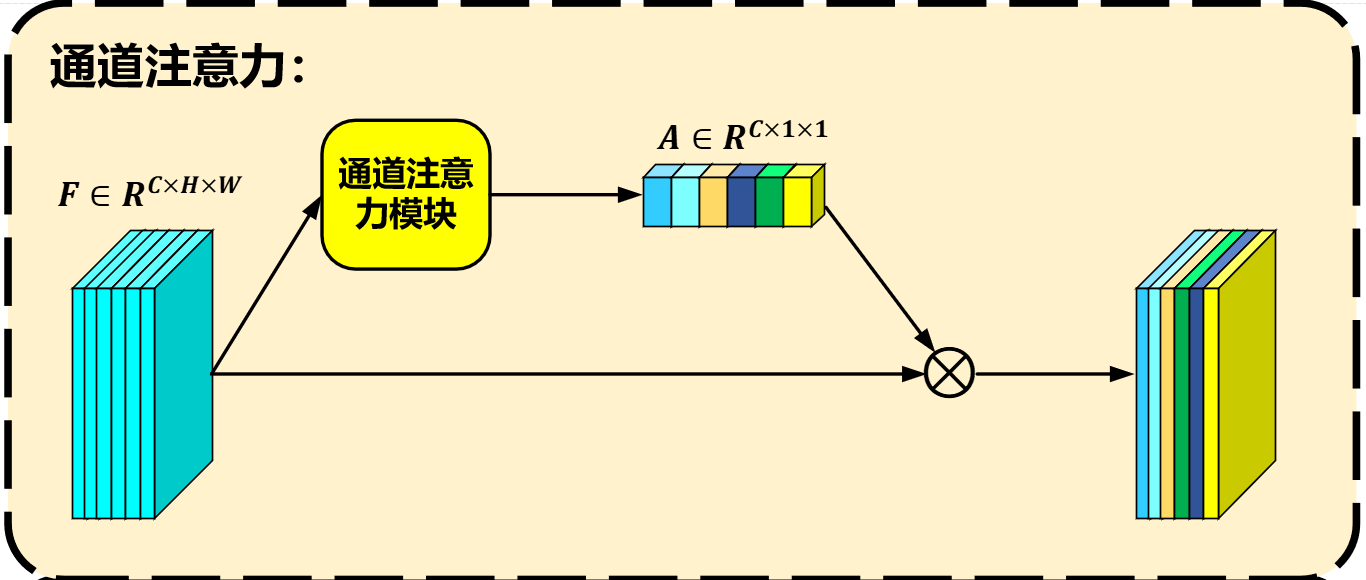SENet提出之后，不少方法对其进行改进，有的简化了特征变换模块、有的改变了融合模式，也有的集成了空间注意力机制。不过，这些方法或多或少都引入了不少参数来学习全局上下文和注意力激活值之间的关系，然而，这样学得的关系也许并不是足够好的。

LCT（linear context transform）观察所得，如下图所示，SE倾向于学习一种负相关，即全局上下文偏离均值越多，得到的注意力激活值就越小。为了更加精准地学习这种相关性，LCT使用一个逐通道地变换来替代SE中的两个全连接层。然而，实验表明，LCT学得的这种负相关质量并不是很高，下图中右侧可以看出，LCT的注意力激活值波动是很大的。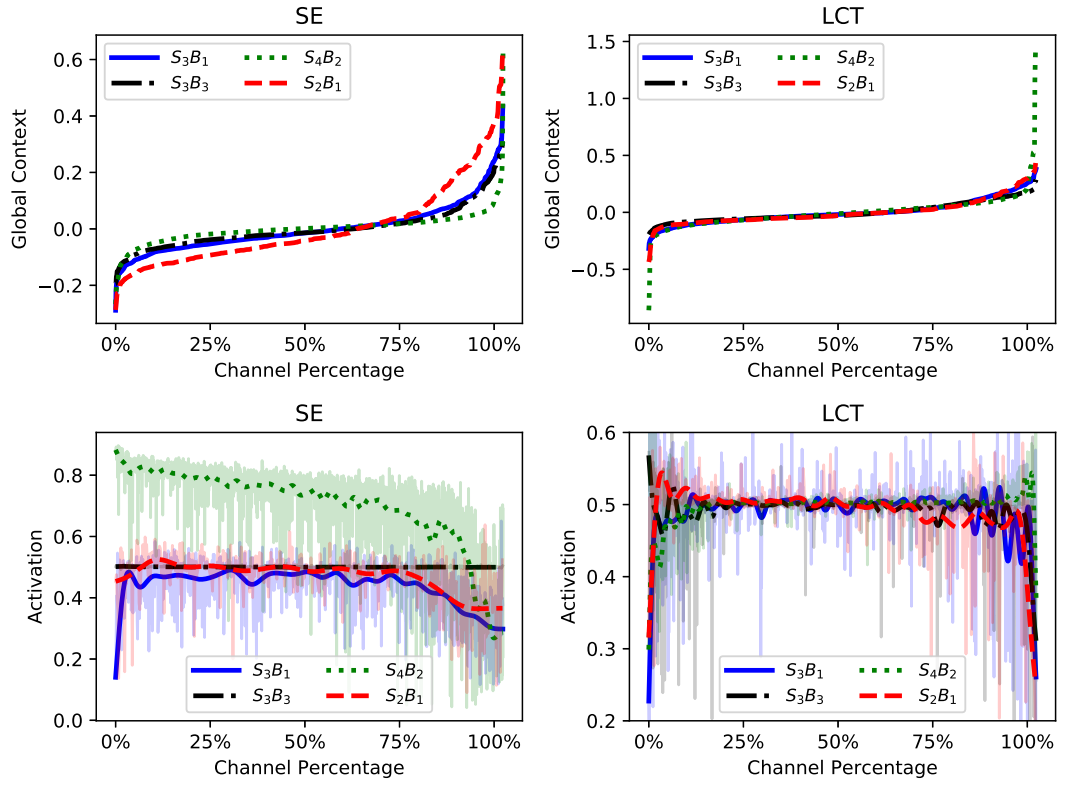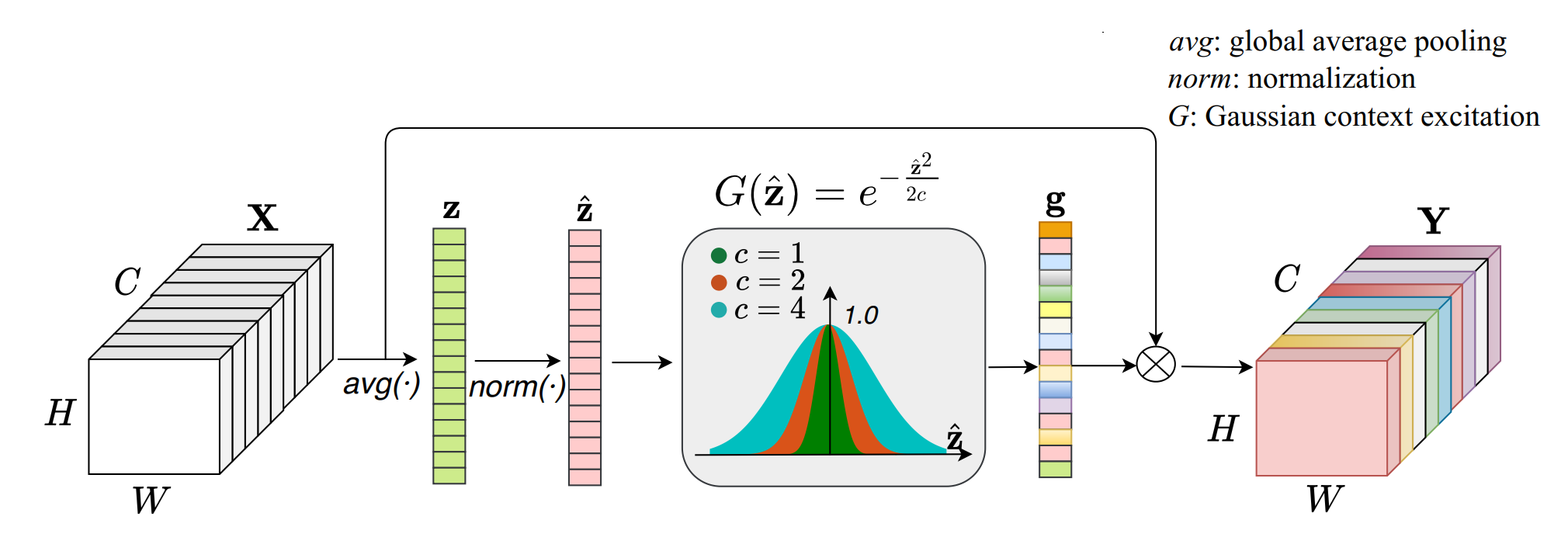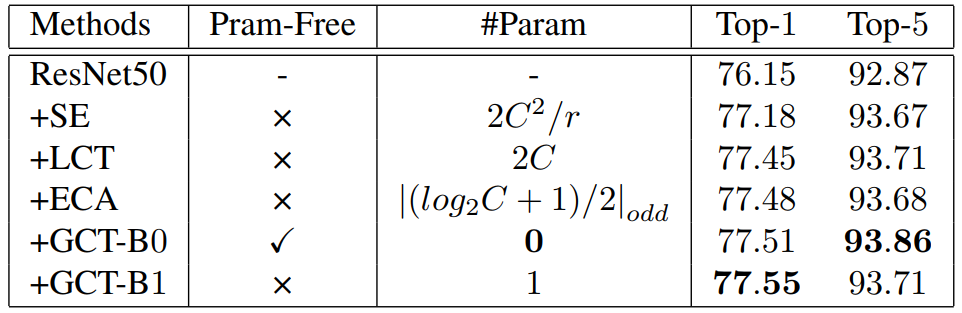## GCT### Gaussian Context Transformer

GCT由三个操作构成，包括全局上下文聚合（global context aggregation，GCA）、标准化（normalization）和高斯上下文激励（gaussian context excitation，GCE），在上图中它是先后进行的。

1. $f(\hat{\mathbf{z}})$的取值范围应在$(0,1]$内才能作为注意力值，即$f(\hat{\mathbf{z}}) \in(0,1]$；
2. 当$\hat{\mathbf{z}}$取值为0的时候，$f(\hat{\mathbf{z}})$取得唯一最大值1；
3. 当$\hat{\mathbf{z}}$小于0时$f(\hat{\mathbf{z}})$单调增加，当$\hat{\mathbf{z}}$大于0时$f(\hat{\mathbf{z}})$单调减少；
4. 有$\lim _{\hat{\mathbf{z}} \rightarrow \pm \infty} f(\hat{\mathbf{z}})=0$。

## 实验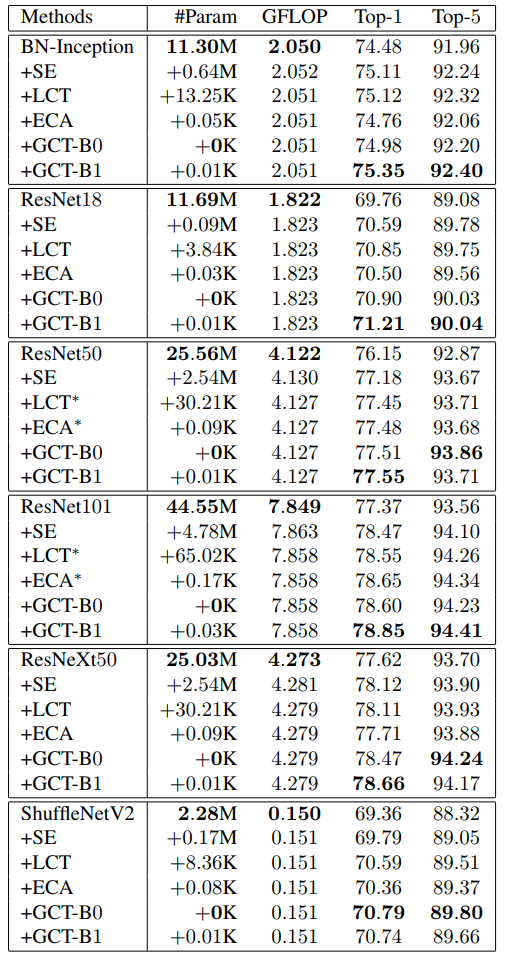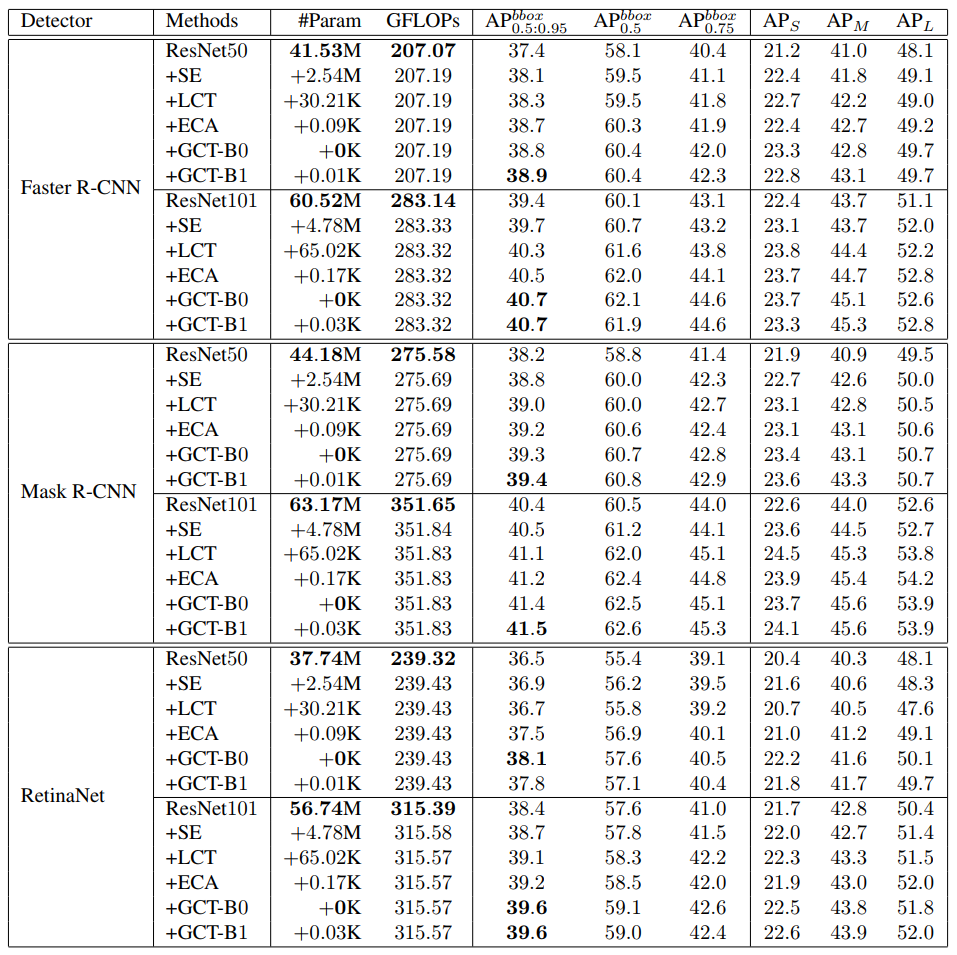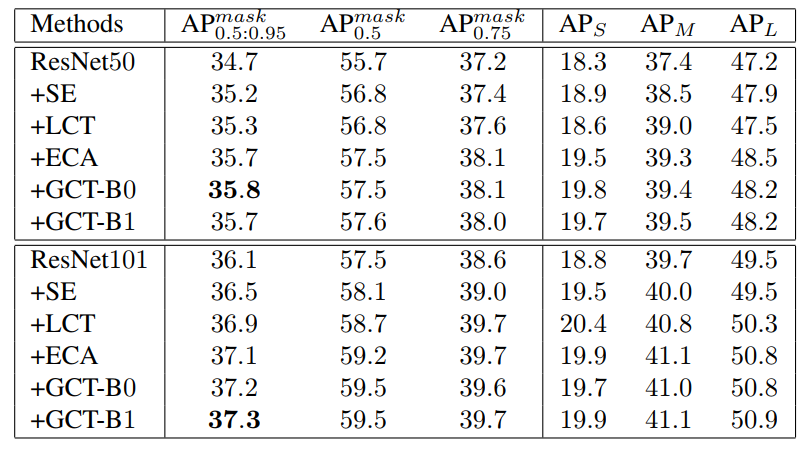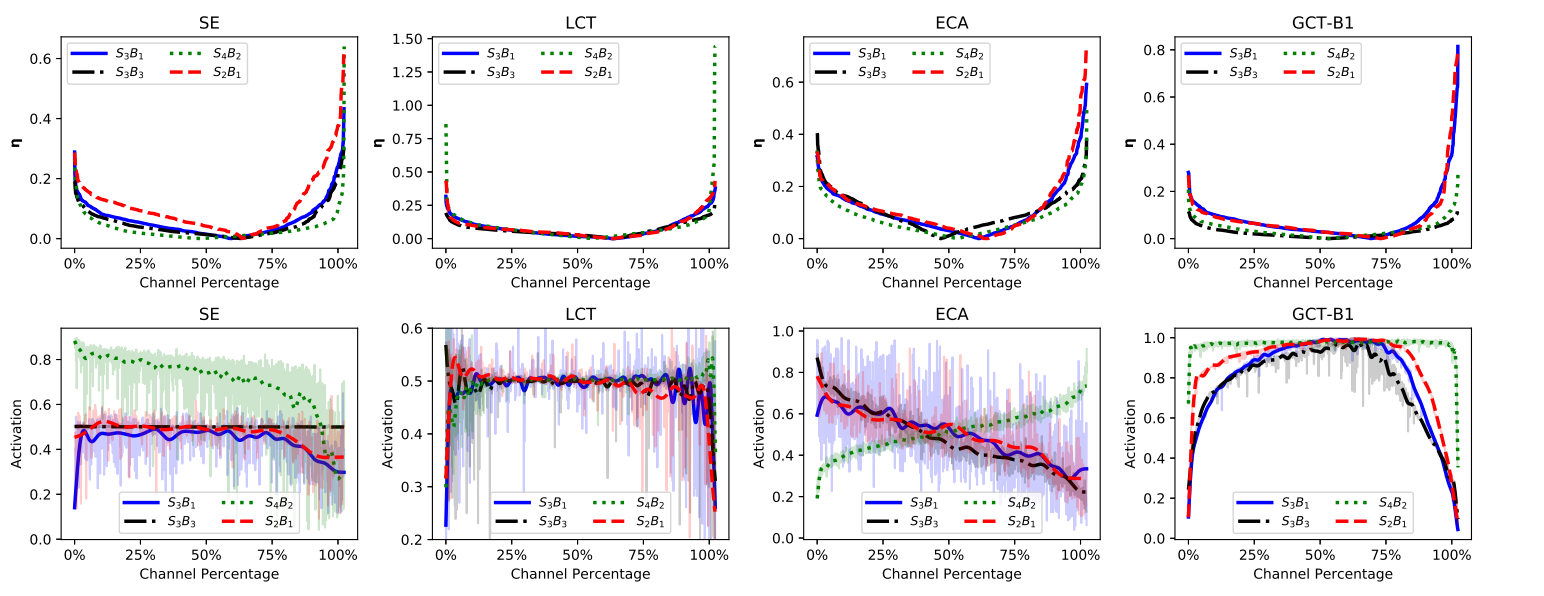目录